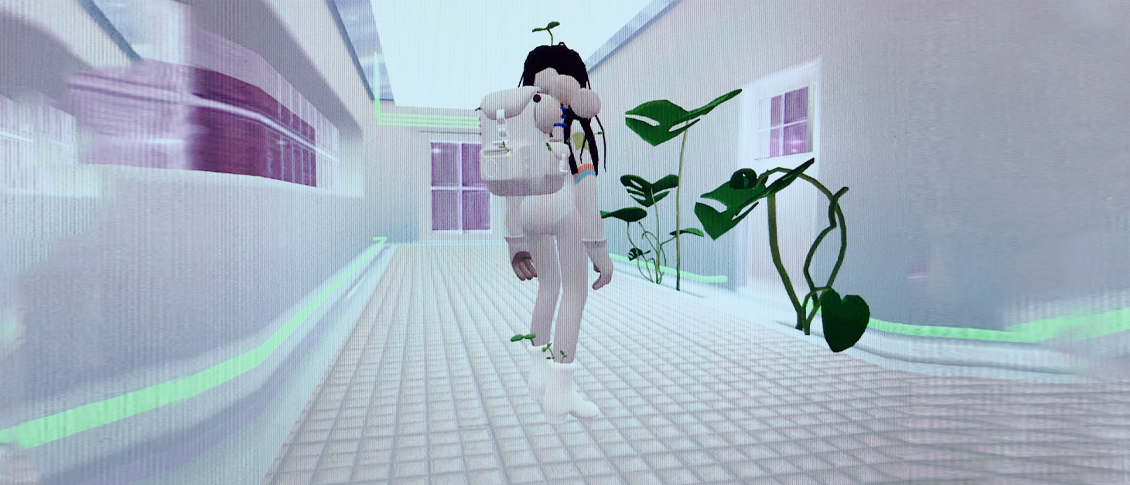✧ﾟ･cinni's game world･ﾟ✧# ✧ scripts ✧

a place to dump my scripts so i have em all in one place...

✧ walk randomly ✧

✧ animated textures✧

## ✧ follow the player ✧

SC_NPCFollow.cs
using UnityEngine;
using UnityEngine.AI;

{
//Transform that NPC has to follow
public Transform transformToFollow;
//NavMesh Agent variable
NavMeshAgent agent;

// Start is called before the first frame update
void Start()
{
agent = GetComponent();
}

// Update is called once per frame
void Update()
{
agent.destination = transformToFollow.position;
}
}

## ✧ walk randomly ✧

(character agent = npc, destination change = empty game object)

CharacterAgent.cs
using System.Collections;
using System.Collections.Generic;
using UnityEngine;
using UnityEngine.AI;

public class CharacterAgent : MonoBehaviour
{
public GameObject characterDesination;
NavMeshAgent theAgent;

void Start()
{
theAgent = GetComponent();
}

void Update()
{
theAgent.SetDestination(characterDesination.transform.position);
}
}

DestinationChange.cs
using System.Collections;
using System.Collections.Generic;
using UnityEngine;

public class DestinationChange : MonoBehaviour
{
public int xPos;
public int zPos;

void OnTriggerEnter(Collider other)
{
if (other.tag == "Character")
{
xPos = Random.Range(312, 559);
zPos = Random.Range(-270, -70);
this.gameObject.transform.position = new Vector3(xPos, 27f, zPos);
}
}

}

## ✧ animated textures ✧

(uses sprite sheets)

AnimatedTexture.cs
using System.Collections;
using System.Collections.Generic;
using UnityEngine;

//AnimatedTexture
public class AnimatedTexture : MonoBehaviour
{

//vars for the whole sheet
public int colCount = 4;
public int rowCount = 4;

//vars for animation
public int rowNumber = 0; //Zero Indexed
public int colNumber = 0; //Zero Indexed
public int totalCells = 4;
public int fps = 10;

//private vars
private Vector2 offset;
private Renderer renderer;

//Start
void Start() { renderer = this.GetComponent(); }

//Update
void Update() { SetSpriteAnimation(colCount, rowCount, rowNumber, colNumber, totalCells, fps); }

//SetSpriteAnimation
void SetSpriteAnimation(int colCount, int rowCount, int rowNumber, int colNumber, int totalCells, int fps)
{

// Calculate index
int index = (int)(Time.time * fps);
// Repeat when exhausting all cells
index = index % totalCells;

// Size of every cell
float sizeX = 1.0f / colCount;
float sizeY = 1.0f / rowCount;
Vector2 size = new Vector2(sizeX, sizeY);

// split into horizontal and vertical index
var uIndex = index % colCount;
var vIndex = index / colCount;

// build offset
// v coordinate is the bottom of the image in opengl so we need to invert.
float offsetX = (uIndex + colNumber) * size.x;
float offsetY = (1.0f - size.y) - (vIndex + rowNumber) * size.y;
Vector2 offset = new Vector2(offsetX, offsetY);

renderer.material.SetTextureOffset("_MainTex", offset);
renderer.material.SetTextureScale("_MainTex", size);
}
}